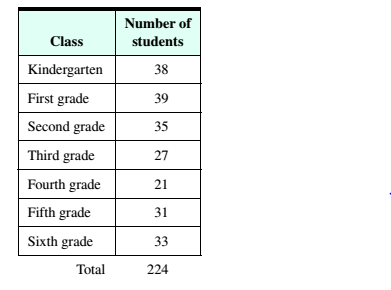# Teacher Aides A total of 25 teacher aides are to be apportioned among seven classes at a new elementary school. The enrollments in the seven classes are shown in the following table. a. Determine the standard divisor. What is the meaning of the standard divisor in the context of this exercise? b. Use the Hamilton method to determine the number of teacher aides to be apportioned to each class. c. Use the Jefferson method to determine the number of teacher aides to be apportioned to each class. Is this apportionment in violation of the quota rule? d. How do the apportionment results produced using the Jefferson method compare with the results produced using the Hamilton method?### Mathematical Excursions (MindTap C...

4th Edition
Richard N. Aufmann + 3 others
Publisher: Cengage Learning
ISBN: 9781305965584

#### Solutions

Chapter
Section### Mathematical Excursions (MindTap C...

4th Edition
Richard N. Aufmann + 3 others
Publisher: Cengage Learning
ISBN: 9781305965584
Chapter 4.1, Problem 2ES
Textbook Problem
1003 views

## Teacher Aides A total of 25 teacher aides are to be apportioned among seven classes at a new elementary school. The enrollments in the seven classes are shown in the following table.a. Determine the standard divisor. What is the meaning of the standard divisor in the context of this exercise?b. Use the Hamilton method to determine the number of teacher aides to be apportioned to each class.c. Use the Jefferson method to determine the number of teacher aides to be apportioned to each class. Is this apportionment in violation of the quota rule?d. How do the apportionment results produced using the Jefferson method compare with the results produced using the Hamilton method?

To determine

(a)

Determine the standard divisor What is the meaning of the standard divisor in the context of this exercise?

### Explanation of Solution

Given Information:

Standard divisor

Concept used:

Standard Divisor=Total PopulationNumber of Representatives

Calculation:

Standard Divisor=Total PopulationNumber of Representatives

To determine

(b)

Use the Hamilton method to determine the number of teacher aides to be apportioned to each class.

To determine

(c)

Use the Jefferson method to determine the number of teacher aides to be apportioned to each class, Is this apportionment in violation of ttie quota rule?

To determine

(d)

How do the apportionment results produced using the Jefferson method compare with the results produced using the Hamilton method?

### Still sussing out bartleby?

Check out a sample textbook solution.

See a sample solution

#### The Solution to Your Study Problems

Bartleby provides explanations to thousands of textbook problems written by our experts, many with advanced degrees!

Get Started

Find more solutions based on key concepts
Evaluate the expression sin Exercises 116. (2)3

Finite Mathematics and Applied Calculus (MindTap Course List)

In Exercises 2336, find the domain of the function. 28. f(x)=x5

Applied Calculus for the Managerial, Life, and Social Sciences: A Brief Approach

Find the exact length of the curve. 16. y=3+12cosh2x,0x1

Single Variable Calculus: Early Transcendentals

True or False: If y = f(x) is not differentiable at a point x = a, then y = f(x) is not continuous at x = a.

Study Guide for Stewart's Single Variable Calculus: Early Transcendentals, 8th

In Problems 3748 find either F(s) or f(t), as indicated. 48. 1{e2ss2(s1)}

A First Course in Differential Equations with Modeling Applications (MindTap Course List)

Construct a stem-and-leaf display for the following data.

Modern Business Statistics with Microsoft Office Excel (with XLSTAT Education Edition Printed Access Card) (MindTap Course List)

Reminder Round all answer to two decimal places unless otherwise indicated. Difference Quotients Calculate the ...

Functions and Change: A Modeling Approach to College Algebra (MindTap Course List)